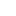### Solution : Potential field created by a disc at a point on its axis of revolution - electrostatic potential - Corrected exercises electromagnetism

1. The potential at a point M of the axis.
Consider a surface area element of the disk centered at a point P. The electrostatic potential created at a point M of the axis Oz is expressed as:
Since r and θ variables are separated :
For the calculation is performed following variable change:
For :
On the other hand :
We get :
Taking the zero potential at infinity is obtained:
2. Field at a point M of the axis.
The z axis is axis of symmetry of the charge distribution. The field at a point M of the axis is carried by this axis:
We must therefore calculate the derivative of the function.

As